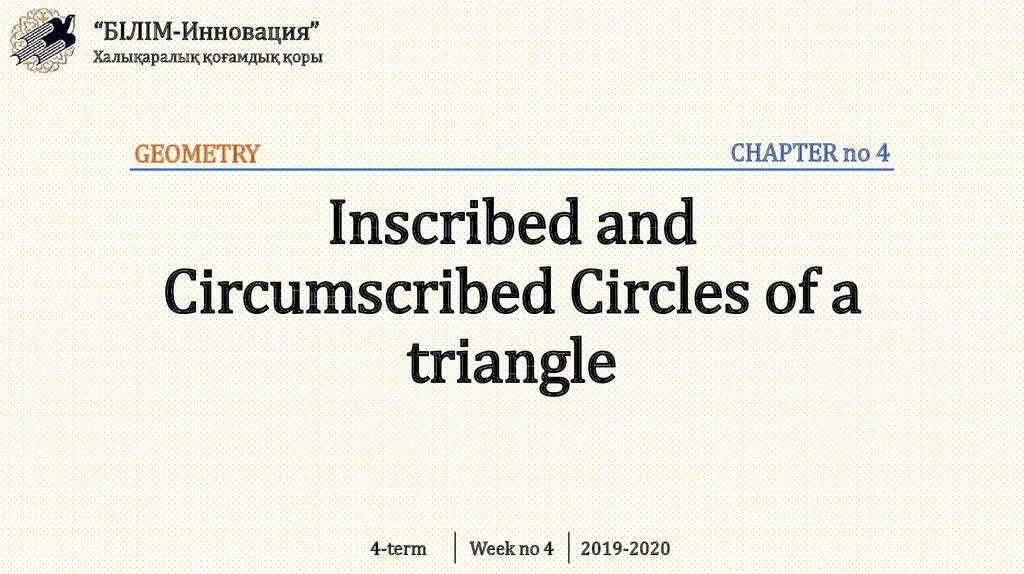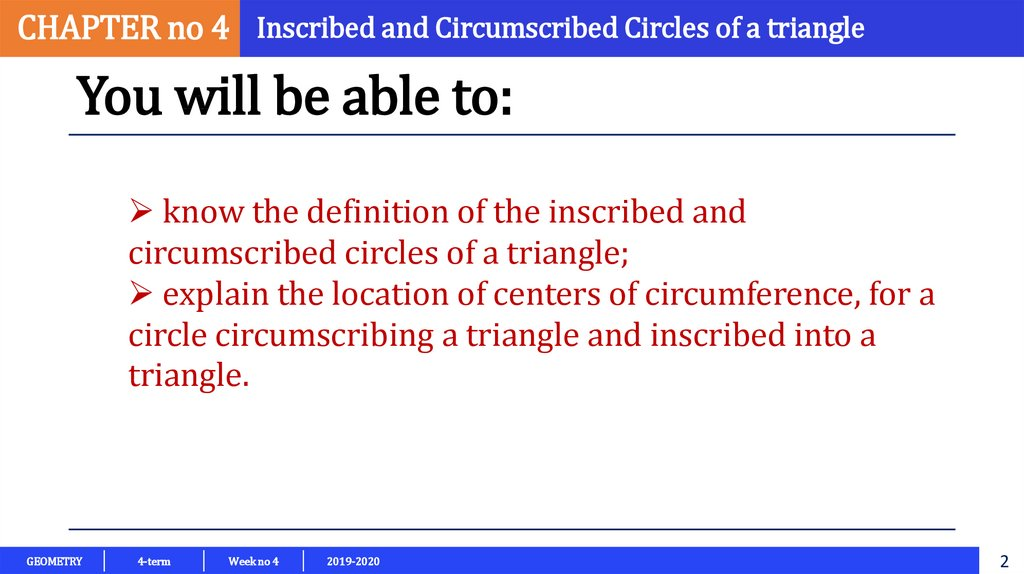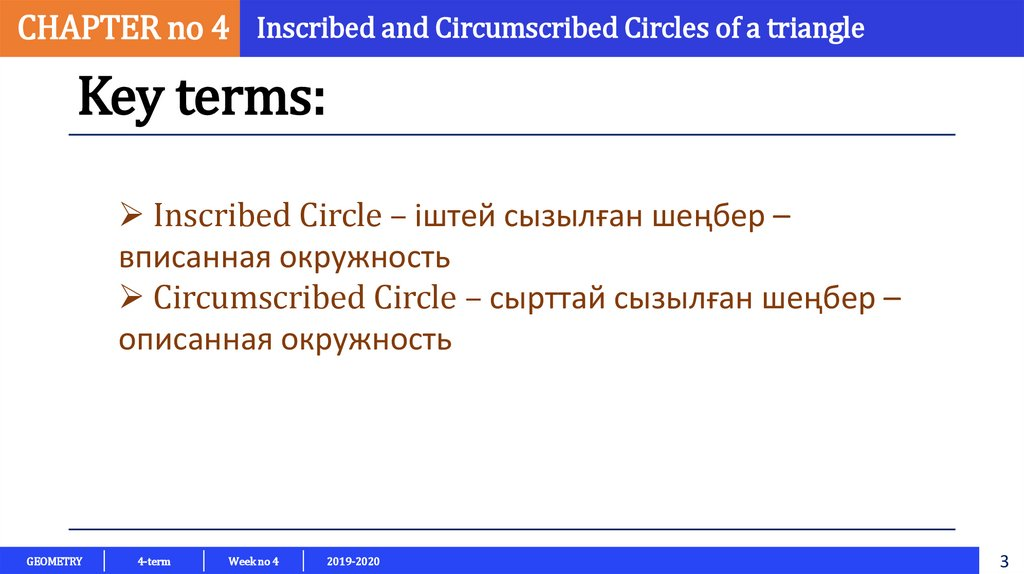Inscribed and circumscribed circles of a triangle

1. Inscribed and Circumscribed Circles of a triangle

“БІЛІМ-Инновация”
Халықаралық қоғамдық қоры
CHAPTER no 4
GEOMETRY
Inscribed and
Circumscribed Circles of a
triangle
4-term
Week no 4
2019-2020

2.

CHAPTER no 4 Inscribed and Circumscribed Circles of a triangle
You will be able to:
know the definition of the inscribed and
circumscribed circles of a triangle;
explain the location of centers of circumference, for a
circle circumscribing a triangle and inscribed into a
triangle.
GEOMETRY
4-term
Week no 4
2019-2020
2

3.

CHAPTER no 4 Inscribed and Circumscribed Circles of a triangle
Key terms:
Inscribed Circle – іштей сызылған шеңбер –
вписанная окружность
Circumscribed Circle – сырттай сызылған шеңбер –
описанная окружность
GEOMETRY
4-term
Week no 4
2019-2020
3

4.

CHAPTER no 4 Inscribed and Circumscribed Circles of a triangle
Review:
Imagine triangle, in this triangle drawn 3 angle
bisectors from each vertices, and intersecting point
of all angle bisectors INCENTER
GEOMETRY
4-term
Week no 4
2019-2020
4

5.

CHAPTER no 4 Inscribed and Circumscribed Circles of a triangle
Definition:
A circle is INSCRIBED in the triangle if triangle’s
three sides are all tangent to the circle.
The center of the circle inscribed in a triangle is
incenter of a triangle.
GEOMETRY
4-term
Week no 4
2019-2020
5

6.

CHAPTER no 4 Inscribed and Circumscribed Circles of a triangle
Example:
Find the center of inscribed circle of a
triangle ABC.
Solution:
As we know, incenter is a center of inscribed circle
of a triangle. Let us use protractor to find half of
measure of each angle. We draw angle bisectors of
triangle ABC, like AM, BK and CH. The point E is a
center of inscribed circle of the triangle ABC
GEOMETRY
4-term
Week no 4
2019-2020
6

7.

CHAPTER no 4 Inscribed and Circumscribed Circles of a triangle
Definition:
A CIRCUMSCRIBED circle of a triangle
is a circle that passes through all the
vertices of the triangle.
A circumcenter of a triangle, the point
where the perpendicular bisectors of
the sides meet.
GEOMETRY
4-term
Week no 4
2019-2020
7

8.

CHAPTER no 4 Inscribed and Circumscribed Circles of a triangle
Example:
Construct the circumcenter of the
triangle ABC.
Solution:
As we know, circumcenter of a triangle is a center of a
circumscribed circle of a triangle. And circumcenter is
intersecting point of perpendicular bisectors of the
sides. At firstly, we find midpoints of the each side of a
triangle using ruler, then, draw a perpendicular line to
midpoint of each side of a triangle.
GEOMETRY
4-term
Week no 4
2019-2020
8

9.

CHAPTER no 4 Inscribed and Circumscribed Circles of a triangle
Draw three triangles of different shapes and then
construct the circle that circumscribes them. Next,
draw three triangles and then construct the circle
that inscribes them.
GEOMETRY
4-term
Week no 4
2019-2020
9

10.

CHAPTER no 4 Inscribed and Circumscribed Circles of a triangle
Resources:
https://www.onlinemathlearning.com/inscribed-circle.html
https://www.onlinemathlearning.com/inscribed-circumscribed-circles-hsg-c3.html
http://www.malinc.se/math/geometry/dothisen.php
GEOMETRY
4-term
Week no 4
2019-2020
10